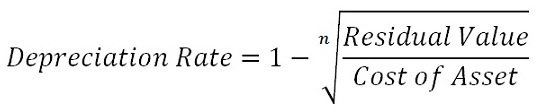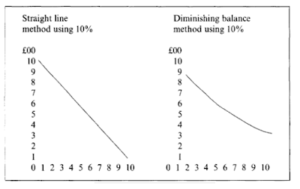# Straight Line Method and Written Down: A Comparative Analysis, Example

The compilation of these Depreciation, Provision and ReservesNotes makes students exam preparation simpler and organised.

## Straight Line Method and Written Down: A Comparative Analysis

The basis of charging depreciation in accounts impacts the amount of profit earned or loss incurred during a year. Thus, it is important to choose the method of charging depreciation wisely, in order to arrive at the correct value of gain or loss. Let us understand how the straight-line method of depreciation is different from the written down value method.

### The Straight Line Method

In this method of depreciation, we write off a fixed value every year during the useful life of the asset. The reason for this being reduction of the value of the asset to zero or the scrap value at the end of the useful life. In this method, we spread the cost of the asset equally over the lifetime of the asset. This method is also known as the fixed installment method.

A particular asset is expected to generate equal utility during its useful life. Let’s understand the formula for calculating the rate of depreciation by the straight-line method:

Depreciation rate = $$\frac{Depreciation}{cost of asset}$$ × 100

### The Written Down Value Method

In this method, a fixed percentage of the reducing balance is written off every year as depreciation. This reduces the fixed asset to its residual value at the end of its working life. This method is also known as the reducing balance or diminishing balance method where the annual charge of depreciation keeps on decreasing every year.

The depreciation charged in the initial years is higher as compared to the subsequent years. According to this method, the value of the asset is not fully extinguished. Let’s understand the formula for calculating the rate of depreciation:How do SLM and WDV Method of Depreciation Compare?Basis for Comparison SLM WDV Meaning In this method of depreciation, the cost of the asset is spread equally over the life years by writing off a fixed amount every year. In this method of depreciation, a fixed rate of depreciation is charged on the book value of the asset, over its useful life. Calculation of depreciation On original cost On the written-down value of the asset. Annual depreciation charge Remains fixed during the useful life. Reduces every year. Value of asset Completely written off Not completely written off Amount of depreciation Initially lower Initially higher Impact of repairs and depreciation on P&L A/c Increasing trend Remains constant

Example:

Question:
Compare the arithmetical difference between SLM and WDV methods of charging depreciation.